# Texture Synthesis and Inpainting using Patch Projections¶

Important: Please read the installation page for details about how to install the toolboxes. $\newcommand{\dotp}{\langle #1, #2 \rangle}$ $\newcommand{\enscond}{\lbrace #1, #2 \rbrace}$ $\newcommand{\pd}{ \frac{ \partial #1}{\partial #2} }$ $\newcommand{\umin}{\underset{#1}{\min}\;}$ $\newcommand{\umax}{\underset{#1}{\max}\;}$ $\newcommand{\umin}{\underset{#1}{\min}\;}$ $\newcommand{\uargmin}{\underset{#1}{argmin}\;}$ $\newcommand{\norm}{\|#1\|}$ $\newcommand{\abs}{\left|#1\right|}$ $\newcommand{\choice}{ \left\{ \begin{array}{l} #1 \end{array} \right. }$ $\newcommand{\pa}{\left(#1\right)}$ $\newcommand{\diag}{{diag}\left( #1 \right)}$ $\newcommand{\qandq}{\quad\text{and}\quad}$ $\newcommand{\qwhereq}{\quad\text{where}\quad}$ $\newcommand{\qifq}{ \quad \text{if} \quad }$ $\newcommand{\qarrq}{ \quad \Longrightarrow \quad }$ $\newcommand{\ZZ}{\mathbb{Z}}$ $\newcommand{\CC}{\mathbb{C}}$ $\newcommand{\RR}{\mathbb{R}}$ $\newcommand{\EE}{\mathbb{E}}$ $\newcommand{\Zz}{\mathcal{Z}}$ $\newcommand{\Ww}{\mathcal{W}}$ $\newcommand{\Vv}{\mathcal{V}}$ $\newcommand{\Nn}{\mathcal{N}}$ $\newcommand{\NN}{\mathcal{N}}$ $\newcommand{\Hh}{\mathcal{H}}$ $\newcommand{\Bb}{\mathcal{B}}$ $\newcommand{\Ee}{\mathcal{E}}$ $\newcommand{\Cc}{\mathcal{C}}$ $\newcommand{\Gg}{\mathcal{G}}$ $\newcommand{\Ss}{\mathcal{S}}$ $\newcommand{\Pp}{\mathcal{P}}$ $\newcommand{\Ff}{\mathcal{F}}$ $\newcommand{\Xx}{\mathcal{X}}$ $\newcommand{\Mm}{\mathcal{M}}$ $\newcommand{\Ii}{\mathcal{I}}$ $\newcommand{\Dd}{\mathcal{D}}$ $\newcommand{\Ll}{\mathcal{L}}$ $\newcommand{\Tt}{\mathcal{T}}$ $\newcommand{\si}{\sigma}$ $\newcommand{\al}{\alpha}$ $\newcommand{\la}{\lambda}$ $\newcommand{\ga}{\gamma}$ $\newcommand{\Ga}{\Gamma}$ $\newcommand{\La}{\Lambda}$ $\newcommand{\si}{\sigma}$ $\newcommand{\Si}{\Sigma}$ $\newcommand{\be}{\beta}$ $\newcommand{\de}{\delta}$ $\newcommand{\De}{\Delta}$ $\newcommand{\phi}{\varphi}$ $\newcommand{\th}{\theta}$ $\newcommand{\om}{\omega}$ $\newcommand{\Om}{\Omega}$

This numerical tour explores texture synthesis and inpainting using patch averaging.

In :
addpath('toolbox_signal')


## Creating a Dictionary of Patches¶

Given an exemplar image, we extract many patch that are our library. We even perform PCA dimensionality reduction to speed up nearest neighbors search.

The main parameter is the width of the patches.

In :
w = 5;


The other parameter is the number of patch in the exemplar dictionary.

In :
q = 1000;


In :
name = 'corral';
n = 128;
M0 = rescale( crop(M0,n) );


We set up larges |(w,w,n-w+1,n-w+1)| matrices representing the X and Y position of the pixel to extract.

In :
p = n-w+1;


location of pixels

In :
[Y,X] = meshgrid(1:p,1:p);


offsets

In :
[dY,dX] = meshgrid(0:w-1,0:w-1);


location of pixels to extract

In :
X = reshape(X, [1 1 p p]);
Y = reshape(Y, [1 1 p p]);
X = repmat(X, [w w 1 1]) + repmat(dX, [1 1 p p]);
Y = repmat(Y, [w w 1 1]) + repmat(dY, [1 1 p p]);


We extract all the patches and reshape the matrix of patch so that |P(:,:,i)| is a patch

In :
P0 = M0(X + (Y-1)*n);
P0 = reshape(P0,w,w,p*p);


Sub-sample the patches

In :
sel = randperm(size(P0,3)); sel = sel(1:q);
P0 = P0(:,:,sel);


## Image Patch-wise Projection¶

The basic step for synthesis or inpainting is to project each patch of an image to its nearest neighbor in the dictionary.

The initial image is just noise for instance.

In :
n = 128;
M = rand(n);


We define an offset vector to shift the projected patch. This needs to be changed during the iteration of the algorithm (either synthesis or inpainting).

In :
ofx = 2;
ofy = 1;


Patch locations.

In :
[Y,X] = meshgrid(1:w:n, 1:w:n);
p = size(X,1);
[dY,dX] = meshgrid(0:w-1,0:w-1);
X = reshape(X, [1 1 p p]);
Y = reshape(Y, [1 1 p p]);
X = repmat(X, [w w 1 1]) + repmat(dX, [1 1 p p]);
Y = repmat(Y, [w w 1 1]) + repmat(dY, [1 1 p p]);


Shift location, with proper boundary condition (cyclic).

In :
Xs = mod(X+ofx-1, n)+1;
Ys = mod(Y+ofy-1, n)+1;


Extract the patches.

In :
P = M(Xs + (Ys-1)*n);


Replace each patch by its closest match.

In :
for i=1:p*p
% distance to current patch
d = sum(sum( (P0 - repmat(P(:,:,i), [1 1 q])).^2 ) );
% best match
[tmp,s] = min(d);
% replace the patch
P(:,:,i) = P0(:,:,s);
end


Reconstruct the image.

In :
Mp = M;
Mp(Xs + (Ys-1)*n) = P;


Display.

In :
clf;
imageplot(M,'Input', 1,2,1);
imageplot(Mp,'Projected', 1,2,2);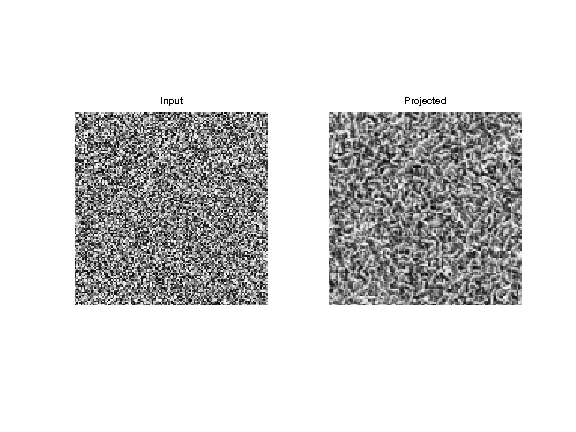## Texture Synthesis¶

Texture synthesis is obtained by performing the projection for several offset and averaging the results.

To speed up performance, we consider only a subset of all possible |w*w| offsets.

In :
noffs = 10;


Compute a randomized list of offsets

In :
sel = randperm(w*w); sel = sel(1:noffs);
OffX = dX(sel); OffY = dY(sel);


We perform one step of synthesis by cycling through the offset.

In :
M1 = zeros(n);
for j=1:noffs
ofx = OffX(j);
ofy = OffY(j);
% shift locations
Xs = mod(X+ofx-1, n)+1;
Ys = mod(Y+ofy-1, n)+1;
% extract patch
P = M(Xs + (Ys-1)*n);
% replace by closest patch
for i=1:p*p
d = sum(sum( (P0 - repmat(P(:,:,i), [1 1 q])).^2 ) );
[tmp,s] = min(d);
P(:,:,i) = P0(:,:,s);
end
% reconstruct the image.
M1(Xs + (Ys-1)*n) = M1(Xs + (Ys-1)*n) + P;
end
M1 = M1 / noffs;


To enhance the synthesis result, we perform histogram equalization.

In :
M1 = perform_hist_eq(M1,M0);


Display the result.

In :
clf;
imageplot(M,'Input', 1,2,1);
imageplot(M1,'Projected', 1,2,2);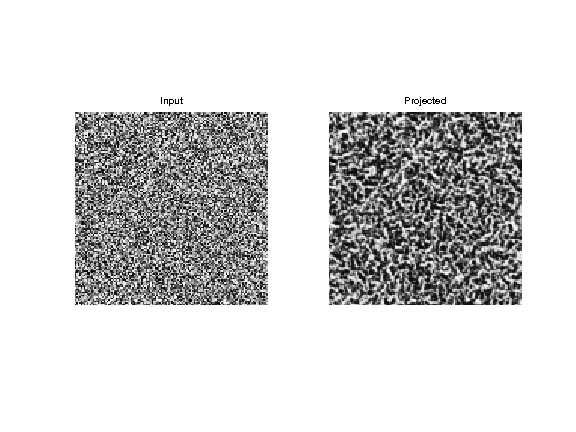Exercise 1

Perform several step of synthesis.

In :
exo1()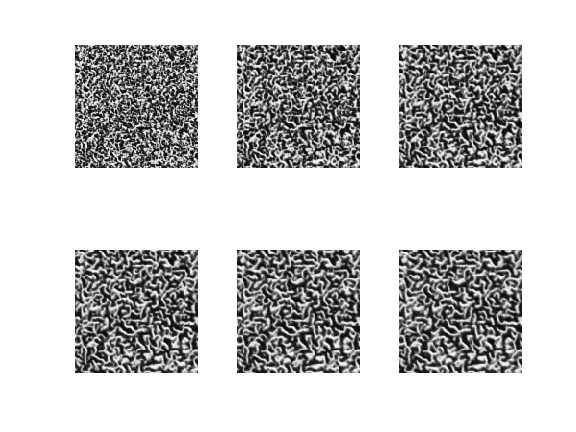In :
%% Insert your code here.


Display the results.

In :
clf;
imageplot(M0,'Exemplar', 1,2,1);
imageplot(M,'Synthesized', 1,2,2);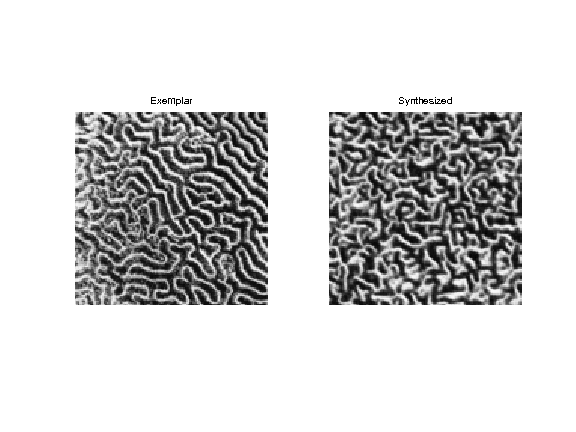Exercise 2

Perform more iteration, and increase the value of |q| and |noffs|.

In :
exo2()

In :
%% Insert your code here.


Exercise 3

Explore the influence of the parameters |w| and |q| on the quality of the synthesis.

In :
exo3()

In :
%% Insert your code here.


Exercise 4

Perform the synthesis using different textures.

In :
exo4()

In :
%% Insert your code here.


Exercise 5

Extend the algorithm to handle color textures.

In :
exo5()

In :
%% Insert your code here.


## Texture Inpainting¶

Texture inpainting corresponds to filling in large hole in an image. It It corresponds to a constraints synthesis inside the area of the hole.

In :
name = 'hair';
n = 128;
Ma = rescale( crop(Ma,n) );


Compute a binary mask representing holes in the textures.

size of the holes

In :
h = 9;


number of holes

In :
nh = 20;
[V,U] = meshgrid(1:n,1:n);
for i=1:nh
% location of the hole
x = floor(rand(2,1)*(n-1))+1;
d = (U-x(1)).^2 + (V-x(2)).^2;
end


Create holes.

In :
M0 = Ma;


Display with / without holes.

In :
clf;
imageplot(Ma, 'Original', 1,2,1);
imageplot(M0, 'To inpaint', 1,2,2);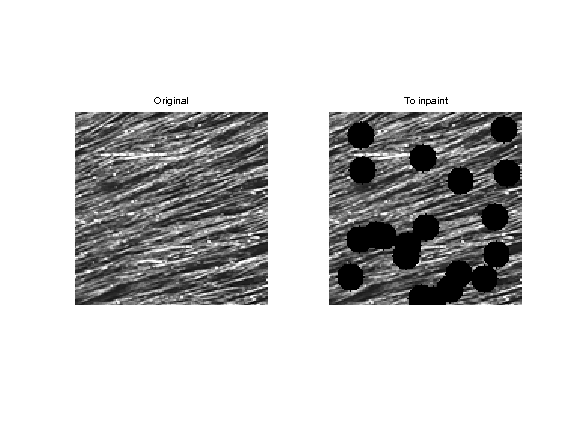Collect all the patches from the image.

In :
p = n-w+1;
[Y,X] = meshgrid(1:p,1:p);
[dY,dX] = meshgrid(0:w-1,0:w-1);
X = reshape(X, [1 1 p p]);
Y = reshape(Y, [1 1 p p]);
X = repmat(X, [w w 1 1]) + repmat(dX, [1 1 p p]);
Y = repmat(Y, [w w 1 1]) + repmat(dY, [1 1 p p]);
P0 = M0(X + (Y-1)*n);
P0 = reshape(P0,w,w,p*p);


Remove those that cross the holes.

In :
I = find( min(min(P0,[],1),[],2)~=0 );
P0 = P0(:,:,I);


Number of patches.

In :
q = 1000;


Sub-sample the patches

In :
sel = randperm(size(P0,3)); sel = sel(1:q);
P0 = P0(:,:,sel);


Initialize the inpainting with random values inside the hole.

In :
M = M0;
M(I) = rand(length(I),1);


Extract non-overlapping patches in the image, with a given offset.

In :
ofx = 2; ofy = 1;
[Y,X] = meshgrid(1:w:n, 1:w:n);
p = size(X,1);
[dY,dX] = meshgrid(0:w-1,0:w-1);
X = reshape(X, [1 1 p p]);
Y = reshape(Y, [1 1 p p]);
X = repmat(X, [w w 1 1]) + repmat(dX, [1 1 p p]);
Y = repmat(Y, [w w 1 1]) + repmat(dY, [1 1 p p]);
Xs = mod(X+ofx-1, n)+1;
Ys = mod(Y+ofy-1, n)+1;
P = M(Xs + (Ys-1)*n);


Replace each patch by its closest match.

In :
for i=1:p*p
% project only a patch crossing the hole.
% distance to current patch
d = sum(sum( (P0 - repmat(P(:,:,i), [1 1 q])).^2 ) );
% best match
[tmp,s] = min(d);
% replace the patch
P(:,:,i) = P0(:,:,s);
end
end


Reconstruct the image.

In :
Mp = M;
Mp(Xs + (Ys-1)*n) = P;


Impose the values of the known pixels.

In :
Mp(mask==0) = M0(mask==0);


Display.

In :
clf;
imageplot(M,'Input', 1,2,1);
imageplot(Mp,'Projected', 1,2,2);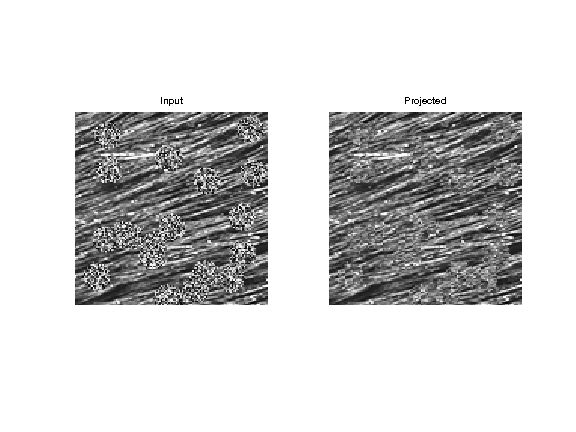Exercise 6

Perform the inpainting by repeating several time the projection with different offsets. You do not needs to average the offset for inpainting. nitialization

In :
exo6()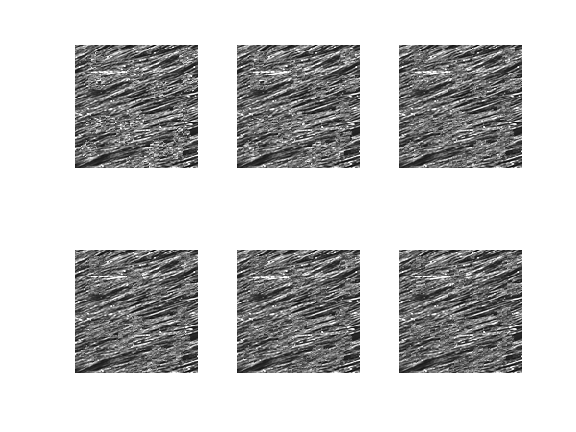In :
%% Insert your code here.


Display.

In :
clf;
imageplot(M0, 'To inpaint', 1,2,1);
imageplot(M, 'Inpainted', 1,2,2);